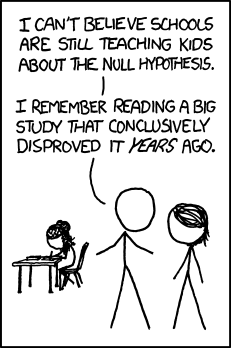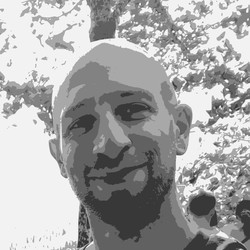# Defending the null hypothesis

A friend was working on a paper and found himself in the situation of having to defend the null hypothesis that a particular effect is absent (or not measurable) when tested under more controlled conditions than those used in previous studies. He asked for some practical advice: “what would convince you as as a reviewer of a null result?”

No statistical test can “prove” a null results (intended as the point-null hypothesis that an effect of interest is zero). You can however: (i) present evidence that the data are more likely under the null hypothesis than under the alternative; or (ii) put a cap on the size of the effect, which could enable you to argue that any effect, if present, is so small that can be considered theoretically or pragmatically irrelevant.

(i) is the Bayesian approach and requires calculating a Bayes factor - that is the ratio between the average (or marginal) likelihood of the data under the null and alternative hypothesis. Note that Bayes factor calculation is highly influenced by the priors (e.g. the prior expectations about the effect size). Luckily, in the case of a single comparison (e.g. a t-test), there is popular way of computing Bayes factors which requires minimal assumptions about the effect of interest, as it is developed using uninformative or minimally informative priors, called the JZW prior (technically correspond to assuming a Cauchy prior on the standardized effect size and a uninformative Jeffrey’s prior on the variances of your measurements). It’s been derived in a paper by Rouder et al. [@Rouder2009c] and there is a easy-to-use R implementation of it in the package BayesFactor, (see function `ttestBF()`).

(ii) is the frequentist alternative. In a frequentist approach you don’t express belief in an hypothesis in terms of probability; uncertainty is characterized in relation to the data-generating process (e.g. how many times you would reject the null if you repeated the experiment a zillion time? - probability is interpreted as the long-run frequency in an imaginary very, very large sample). Under this approach you can estimate what is the maximum size of the effect since you did not detected it in your current experiment. Daniel Lakens has written an easy-to-use package for that, called TOSTER; see this vignette for an introduction.